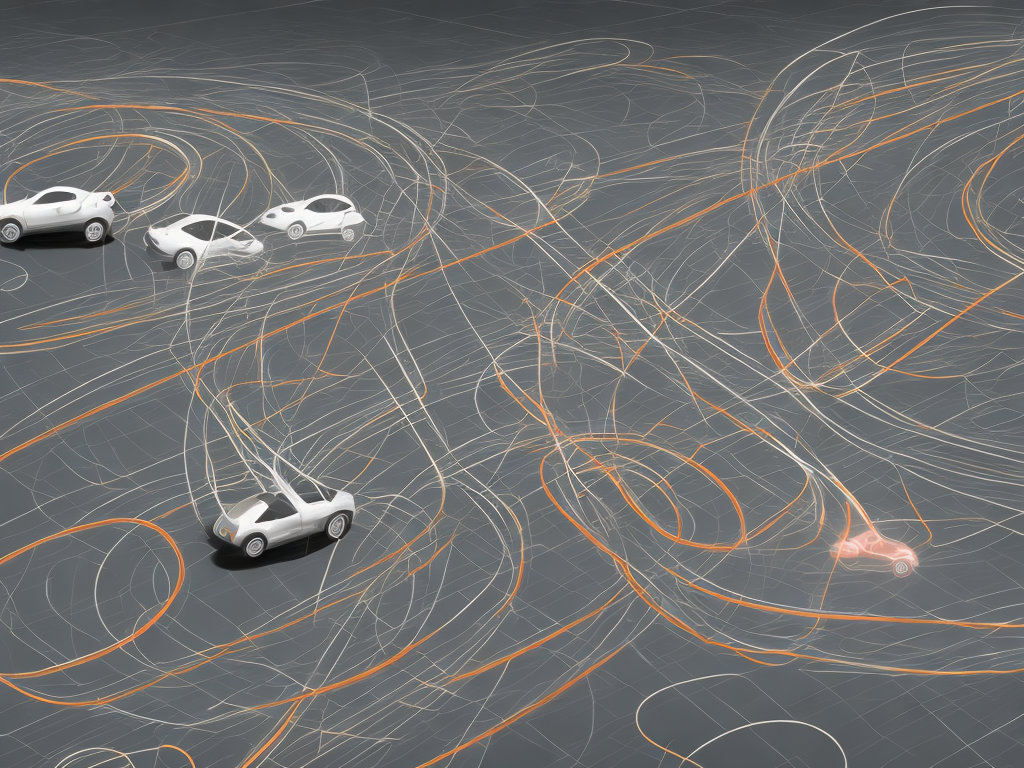# Difference Between Speed And Velocity

tl;dr
Speed is a scalar quantity that measures how fast an object is moving, while velocity is a vector quantity that measures both the speed and direction of an object's motion.Speed and velocity, two terms often used interchangeably, are actually two different concepts in motion physics. Both describe how quickly object is moving or travelling, but there is a crucial difference that sets them apart.

Speed is a scalar quantity that refers to "how fast" an object is moving. It is the rate at which distance is covered by an object in a given time. It is represented by a single numerical value, typically measured in meters per second (m/s), kilometers per hour (km/h), or miles per hour (mph).

On the other hand, velocity is a vector quantity that takes into account not only the speed of an object but also its direction of motion. In other words, velocity is the rate at which an object moves in a specific direction. It is represented by a vector, which specifies both the speed and the direction of the object's motion.

To better understand the difference between speed and velocity, let's consider a simple example. Imagine you are driving a car on a straight road. If you maintain a constant speed of 60 km/h, your speed will remain constant throughout your journey. However, if you turn your steering wheel to the left or right, your direction changes, and so does your velocity. Even though your speed remains constant, your velocity changes because the direction of your motion has changed.

Another example that highlights the difference between speed and velocity is a ball thrown straight vertically upwards. At the highest point, the ball has zero velocity, but it still has a speed because it moved through a distance, which is the maximum height reached. In physics, the term "magnitude" is often used to refer to the value of a scalar quantity such as speed, whereas "magnitude and direction" refers to the value of a vector quantity such as velocity.

Uses and applications of speed and velocity

Speed and velocity are used in different areas of physics, and they have specific applications based on the type of motion involved. For instance, speed is fundamental in kinematics, which is the study of motion without taking into account the forces causing the motion. Kinematics describes the exact motion of an object in terms of its speed and acceleration, without considering the causes of the motion.

Velocity, on the other hand, is used in vector calculus to describe the motion of an object in relation to its environment. More specifically, it is used in dynamics, which is the study of motion considering the forces applied to the object. Velocity is essential to determine how forces affect an object's motion and how the object responds to those forces.

In addition to these physics-specific applications, speed and velocity have a wide range of real-world applications. For example, speed is crucial in industries such as automotive, aerospace, and robotics, where the speed of an object can significantly impact its performance. It is also important in sports such as athletics, cycling, and skiing, where athletes aim to maximize their speed to achieve better results.

Velocity, on the other hand, is more commonly used in navigation, where the direction of a moving object is as important as its speed. The global positioning system (GPS), for instance, relies on velocity measurements to track the movement of objects such as aircraft and ships. Velocity is also used in engineering, particularly in the design and analysis of systems such as automated guided vehicles (AGVs) and drones, where precise movements are essential.

Calculating speed and velocity

Calculating speed and velocity involves different methods, depending on the type of motion involved. In general, speed is calculated by dividing the distance covered by an object by the time taken to cover that distance. The formula for speed is:

Speed = Distance / Time

For example, if a car travels 200 km in 4 hours, its speed is calculated as follows:

Speed = 200 km / 4 hours = 50 km/h

Velocity, on the other hand, involves the displacement of an object rather than the distance it covers. Displacement is the change in position of an object from its initial position to its final position. The formula for velocity is:

Velocity = Displacement / Time

For example, if a car starts at position A and ends at position B, which are 200 km apart, and takes 4 hours to make the journey, its velocity is calculated as follows:

Velocity = (200 km - 0 km) / 4 hours = 50 km/h

In this scenario, the velocity of the car is the same as its speed because the car moved in a straight line from point A to point B.

Conclusion

In summary, speed and velocity are two different concepts in motion physics. Speed is a scalar quantity that refers to "how fast" an object is moving, whereas velocity is a vector quantity that takes into account the speed and direction of motion. Both speed and velocity have different applications in various fields and industries and can be calculated using different formulas based on the type of motion involved. Understanding the difference between these two concepts is essential in grasping the fundamental principles of motion physics.